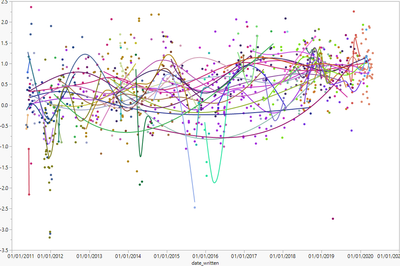Choose Language Hide Translation Bar

## Option for Smoother variable line thickness based off data volume (thicker line for larger N, thinner line for smaller N)

Problem statement: I will often plot a time trend, setting a categorical variable to Overlay role (and often checking several different factors, so first overlaying by column A, then overlay by column B, then column C, etc.) But when there are categories containing many different groups, the plots can get extremely busy (see below,) and it's hard to tell "large N" smoother lines that have a large data set in that group from "small N" lines that are just a spline fit to ~3-5 data points.

Suggestion: Could a "dynamic width" option be added to Smoother that adjusts the line thickness depending on data volume? Lines with a large number of data points would be thicker, and lines with only a few data points would be very thin.

Ideally, the data "density" could also be taken into account: if there are only 2 data points from group X in 2015, but 30 data points in 2016 and 100 in 2017, the group X smoother line thickness would be thin in 2015 and thicker in 2017. (Basically the line thickness would be inversely proportional to the time delta between points - but smoothed so the thickness of the line doesn't become infinite if the time delta between two points gets very close to 0.)

Example problem: This is the kind of "busy plot" I'd like to use this feature with: if the smoother lines with lots of data were very thick/bold and the smoother lines with just a handful of data points were very thin, it would be much easier to see what is a real signal. (There are real signals in this data, I promise.)I hope this is clear enough, please let me know if any clarification is needed.